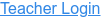• Polynomials
• Factors
• Products

### Description

Build rectangles of various sizes and relate multiplication to area. Discover new strategies for multiplying algebraic expressions. Use the game screen to test your multiplication and factoring skills!

### Sample Learning Goals

• Develop and justify a method to use the area model to determine the product of a monomial and a binomial or the product of two binomials.
• Factor an expression, including expressions containing a variable.
• Recognize that area represents the product of two numbers and is additive.
• Represent a multiplication problem as the area of a rectangle, proportionally or using generic area.
• Develop and justify a strategy to determine the product of two multi-digit numbers by representing the product as an area or the sum of areas.
Version 1.0.0